# matplotlib库学习 Exercise 11.2: Data Exercise 11.3: Histogram and density estimationLearning matplotlib, a lib to draw chart, figure, etc..

Plot the function
\$\$ f(x) = sin^2(x-2)e^{-x^2} \$\$
over the interval [0, 2]. Add proper axis labels, a title, etc.

## Result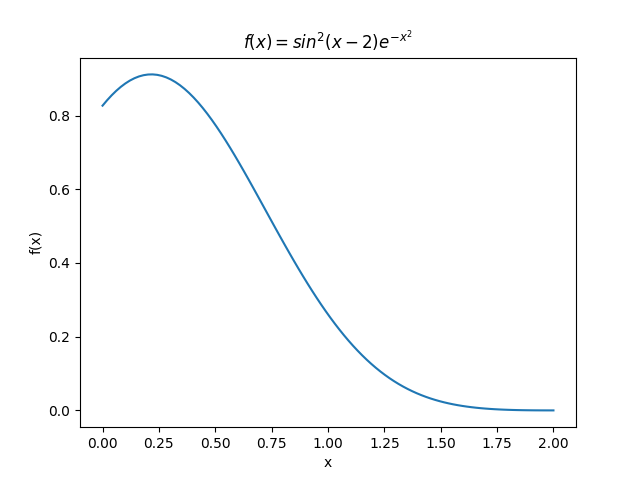# Exercise 11.2: Data

Create a data matrix X with 20 observations of 10 variables. Generate a vector b with parameters. Then generate the response vector \$y = Xb+z\$ where z is a vector with standard normally distributed variables.

Now (by only using y and X), find an estimator for b, by solving
\$\$ hat{b} = argminlimits_{b} || Xb-y ||_2 \$\$
Plot the true parameters b and estimated parameters b̂. See Figure 1 for an example plot.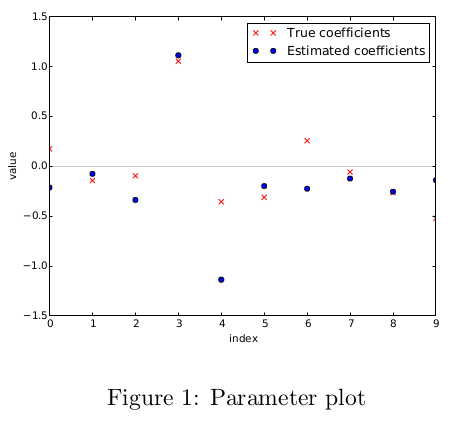## Result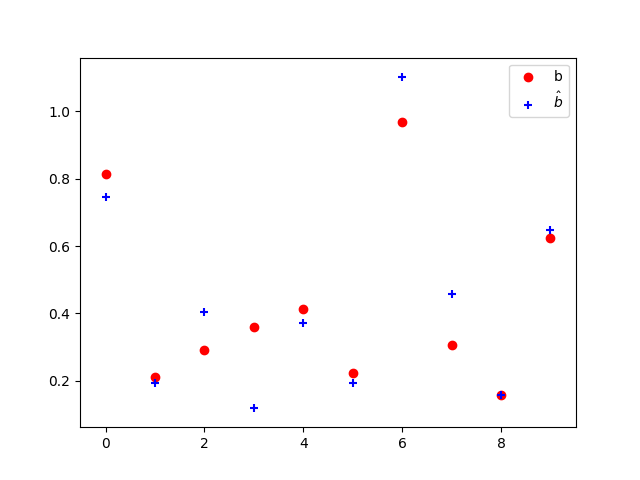## Knowledge

• `.dot()`点乘
• `lstsq()`最小二乘法
• `scatter`散点图
• `legend`显示图例

# Exercise 11.3: Histogram and density estimation

Generate a vector z of 10000 observations from your favorite exotic distribution. Then make a plot that
shows a histogram of z (with 25 bins), along with an estimate for the density, using a Gaussian kernel
density estimator (see scipy.stats). See Figure 2 for an example plot.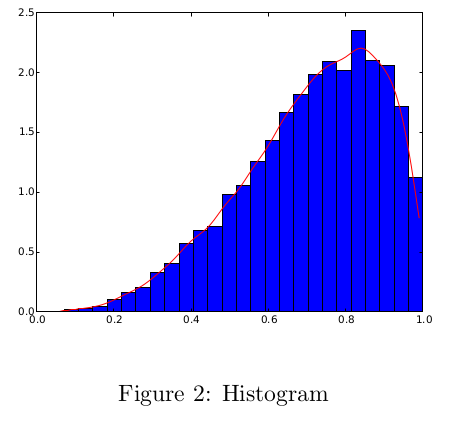## Result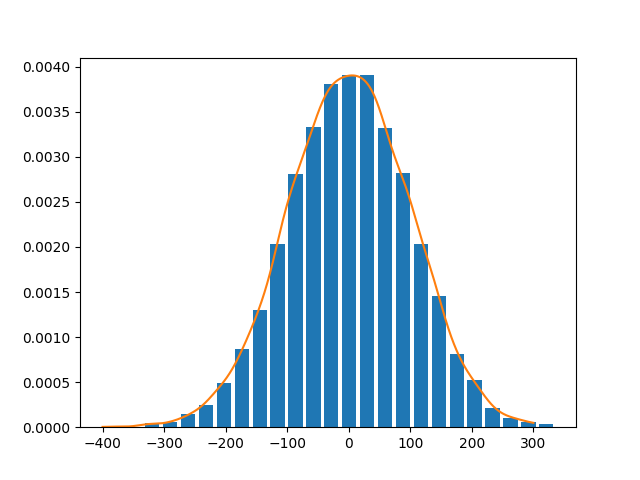## Knowledge

• `hist` to draw a histogram
• `scipy.stats.gaussian_kde` to create Gaussian kernel density estimator.